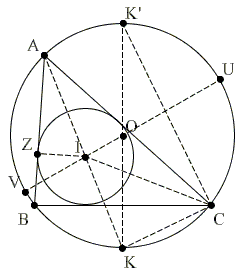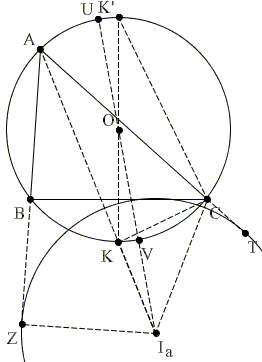# Euler's Formula and Poncelet Porism

Euler's formula that relates the circumradius, the inradius and the distance between the circumcenter and the incenter of a triangle serves the basis for the Poncelet porism for triangles.

The circumradius $R,$ the inradius $r$ and the distance between the circumcenter and the incenter $d$ of a triangle stand in an elegant relationship

$\displaystyle\frac{1}{R - d} + \frac{1}{R + d} = \frac{1}{r},$

which we shall prove in the form

$(1)\qquad 2Rr = R^{2} - d^{2}.$

Let $ABC$ be a triangle with circumcenter $O$ and incenter $I.$ Extend $AI$ to meet the circumcircle the second time at $K.$ Extend $OK$ and $OI$ to obtain two diameters $UV$ and $KK'$ of the circumcricle.By the Intersecting Chords Theorem,

$(2)\qquad AI\cdot IK = IU\cdot IV = (R + d)(R - d) = R^{2} - d^{2}.$

Next, in $\Delta ICK,$ $\angle CIK$ is external to $\Delta ACI.$ Thus, if angles of $\Delta ABC$ are denoted $\alpha,$ $\beta,$ and $\gamma,$ we see that

$\angle CIK = (\alpha + \gamma )/2.$

On the other hand,

\begin{align} \angle ICK &= \angle BCI + \angle BCK \\ &= \gamma /2 + \angle BAK \\ &= \gamma /2 + \alpha /2. \\ \end{align}

It follows that $\angle CIK = \angle ICK$ making $\Delta ICK$ isosceles so that

$(3)\qquad IK = KC.$

Let $Z$ be the point of tangency of $AB$ and the incircle. $\Delta AIZ$ is right with angle at $A$ equal $\alpha /2.$ $\Delta CKK'$ is also right with angle at $K'$ equal $\angle CAK = \alpha /2.$ The two triangles are similar, from which we get a proportion:

$AI / IZ = KK' / KC.$

This is the same as

$(4)\qquad AI\cdot KC = IZ\cdot KK' = r\cdot 2R.$

The combination of (2), (3), and (4) yields (1). (Elsewhere there is a different proof of the formula.)

Note that a similar result holds for excircles, for example,

$(5)\qquad 2Rr_{a} = d_{a}^{2} - R^{2},$

where $r_{a}$ is the $A$-excircle and $d_{a}$ the distance between the circumcenter and the $A$-excenter.The proof must be slightly modified. For example, instead of the Intersecting Chords Theorem, we make use of the Intersecting Secants Theorem.

\begin{align} (d_{a} + R)(d_{a} - R) &= I_{a}U \cdot I_{a}V \\ &= I_{a}A \cdot I_{a}K \\ &= I_{a}A \cdot KC \\ &= (I_{a}Z \cdot KK'/KC) \cdot KC \\ &= r_{a} \cdot 2R. \end{align}

The identity can be written in an elegant way:

$(6)\qquad \displaystyle\frac{1}{d - R} - \frac{1}{d + R} = \frac{1}{r}.$

$II_{a}^{2} = 4R(r_{a} - r),$ and
$I_{b}I_{c}^{2} = 4R(r_{b} + r_{c}).$

To see that, apply the formula for the length of a median to triangles $OII_{a}$ and $OI_{b}I_{b}:$

$OI^{2} + OI_{a}^{2} = 2 OK^{2} + II_{a}^{2}/2,$ and
$OI_{b}^{2} + OI_{c}^{2} = 2 OK'^{2} + I_{b}I_{c}^{2}/2.$

and use the just derived formulas for $OI^{2},$ $OI_{a}^{2},$ and similar.By reversing the argument, we can establish Poncelet porism for triangles.

#### Poncelet Porism

If the distance $d$ between the centers of two circles $O(R)$ and $I(r)$ satisfies (1), an infinite number of triangles may be circumscribed about the circle $I(r)$ which also be inscribed in the circle $O(R).$

Indeed, from any point $A$ on $O(R)$ draw two chords $AB$ and $AC$ tangent to the circle $I(r).$ The line $AI$ bisects angle $BAC,$ and it follows from (1) that

$AI \cdot IK = 2Rr.$

From similar triangles $AIZ$ and $CKK',$

$AI \cdot CK = 2Rr,$

and therefore $IK = KC$ making $I$ the incenter of $\Delta ABC.$

Note: in a similar manner, (6) insures that a porism exists also in case where the incircle is replaced by an excircle.

### References

1. N. Altshiller-Court, College Geometry, Dover, 1980, pp. 85-87
2. J. Casey, A Sequel to Euclid, Scholarly Publishing Office, University of Michigan Library (December 20, 2005), reprint of the 1888 edition, pp. 107-110
3. J. L. Coolidge, A Treatise On the Circle and the Sphere, AMS - Chelsea Publishing, 1971, p. 45
4. F. G.-M., Exercices de Géométrie, Éditions Jacques Gabay, sixiéme édition, 1991, pp. 837-839
5. R. A. Johnson, Advanced Euclidean Geometry (Modern Geometry), Dover, 1960, p. 172
6. T. Lalesco, La Géométrie du Triangle, Éditions Jacques Gabay, sixiéme édition, 1987#### Poncelet Porism